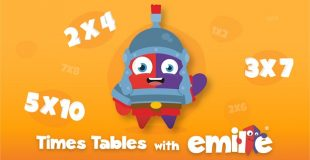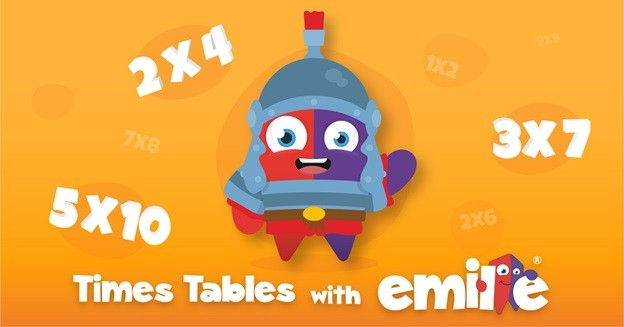### Understanding Fractions in a Relatively Easy Manner

•Do you wonder about fractions? It has been a way of describing a part of a whole. For instance, in case, you have the complete pizza and your friend consumes half of it, he or she would have consumed part of the entire pizza. You would be required to divide the pizza into several pieces as you deem fit. However, every piece would be representing one part of that entire pizza.

You should rest assured that gaining knowledge on how to understand and make use of fractions has been deemed an important skill in both mathematics and routine life.## Defining a fraction

Let us delve on the easy way of understanding the fractions.

### Identifying the numerator

It would be pertinent to mention here that a fraction would always be written with one number over the other number while being divided by a line. The top number would be the numerator of a fraction. It would be best described as a part of the whole that we talked at the beginning of the post. A good example would be in a fraction 1/4, the number ‘1’ would be the numerator. It would imply one part of the whole that entails four parts.

### Identifying the denominator

The denominator would be the number listed below the line in a fraction. It would be representing the whole. The denominator would be best described as the number of parts that would be divided into the whole. A good way to remember the denominator would be taking the alphabet-‘d’ should be referred to as ‘down’ in the denominator. The fraction 1/4 would have ‘4’ as the denominator. It would be the whole divided into four equal parts.

### How to recognize an improper fraction

Only that fraction would be deemed as improper if the numerator has been larger than the denominator. In other words, the top number should be larger than the bottom number. In the fractions, you should not come up with an improper fraction as a final solution. It would be imperative to simplify the fraction into a whole or mixed number.### Simplifying improper fractions into whole or mixed numbers

Most fractions could be simplified and divided into a whole number. However, there would be few fractions that may not be divided in an even manner. The numbers in such fractions would not be divided evenly. Therefore, it should be written as mixed numbers.

It would not be wrong to state that people have been making use of fractions in their routine life. However, it would be identified as decimals.

Do you wonder about fractions? It has been a way of describing a part of a whole. For instance, in case, you have the complete pizza and your friend consumes half of it, he or she would have consumed part of the entire pizza. You would be required to divide the pizza into several pieces…